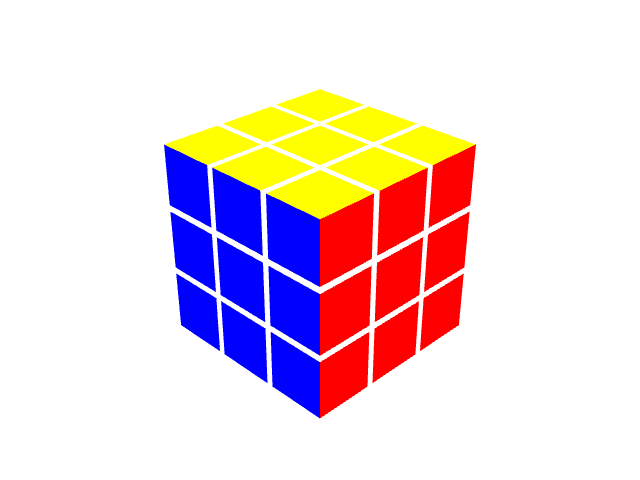## 基本概念

• 位于魔方各角的块称为角块，每个角块均具有 3 个颜色。一个立方体有 8 个角，故而一个魔方也具有 8 个角块。
• 位于魔方各棱上的块称为棱块，每个棱块均具有 2 个颜色。一个立方体有 12 条棱，故而一个魔方也具有 12 个棱块。
• 位于魔方各面中心的块称为中心块，每个中心块仅有 1 个颜色。一个立方体有 6 个面，故而一个魔方也具有 6 个中心块。
• 位于整个魔方中心的块没有颜色，在渲染和还原的过程中也不起到什么实际的用处，我们可以忽略这个块。

## 旋转变换

``````rotate (center, clockwise = true) {
const axis = center.indexOf(1) + center.indexOf(-1) + 1
// Fix y direction in right-handed coordinate system.
clockwise = center !== 0 ? !clockwise : clockwise
// Fix directions whose faces are opposite to axis.
clockwise = center[axis] === 1 ? clockwise : !clockwise

let cs = [[1, 1], [1, -1], [-1, -1], [-1, 1]] // corner coords
let es = [[0, 1], [1, 0], [0, -1], [-1, 0]] // edge coords
const prepareCoord = coord => coord.splice(axis, 0, center[axis])
cs.forEach(prepareCoord); es.forEach(prepareCoord)
if (!clockwise) { cs = cs.reverse(); es = es.reverse() }

// 移动每个块到其新位置
const rotateBlocks = ([a, b, c, d]) => {
const set = (a, b) => { for (let i = 0; i < 6; i++) a[i] = b[i] }
const tmp = []; set(tmp, a); set(a, d); set(d, c); set(c, b); set(b, tmp)
}
const colorsAt = coord => this.getBlock(coord).colors
rotateBlocks(cs.map(colorsAt)); rotateBlocks(es.map(colorsAt))

// 调整每个块的自旋朝向
const swap = [
[[F, U, B, D], [L, F, R, B], [L, U, R, D]],
[[F, D, B, U], [F, L, B, R], [D, R, U, L]]
][clockwise ? 0 : 1][axis]
const rotateFaces = coord => {
const block = colorsAt(coord)
;[block[swap], block[swap], block[swap], block[swap]] =
[block[swap], block[swap], block[swap], block[swap]]
}
cs.forEach(rotateFaces); es.forEach(rotateFaces)
return this
}
``````

## WebGL 渲染基础

1. 初始化着色器（编译供 GPU 执行的程序）
2. 向缓冲区中传递顶点和颜色数据（操作显存）
3. 设置用于观察的透视矩阵和模-视变换矩阵（传递变量给 GPU）
4. 调用 `drawElements``drawArray` 渲染一帧

## 旋转动画实现

• 在一次某个面的旋转过程中，魔方的数据模型不发生改变，仅改变受影响的顶点所在位置。
• 在旋转结束时，我们调用上文中实现的 `rotate` API 来「瞬间旋转好」魔方的数据模型，而后再多绘制一帧。

``````render (rX = 0, rY = 0, moveFace = null, moveAngle = 0) {
if (!this.gl) throw new Error('Missing WebGL context!')
this.buffer = getBuffer(this.gl, this.blocks, moveFace, moveAngle)
renderFrame(this.gl, this.programInfo, this.buffer, rX, rY)
}
``````

``````animate (move = null, duration = 500) {
if (move && move.length === 0) return Promise.resolve()
if (!move || this.__ANIMATING) throw new Error('Unable to animate!')

this.__ANIMATING = true
let k = move.includes("'") ? 1 : -1
if (/B|D|L/.test(move)) k = k * -1
const beginTime = +new Date()
return new Promise((resolve, reject) => {
const tick = () => {
const diff = +new Date() - beginTime
const percentage = diff / duration
const face = move.replace("'", '')
if (percentage < 1) {
this.render(this.rX, this.rY, face, 90 * percentage * k)
window.requestAnimationFrame(tick)
} else {
this.move(move)
this.render(this.rX, this.rY, null, 0)
this.__ANIMATING = false
resolve()
}
}
window.requestAnimationFrame(tick)
})
}
``````

## 连续旋转实现

``````if (Array.isArray(move) && move.length > 1) {
const lastMove = move.pop()
// 返回递归得到的 Promise
return this.animate(move).then(() => this.animate(lastMove))
} else if (move.length === 1) move = move // 继续已有逻辑
``````

## 魔方的还原

1. 还原底部四个棱块，构建出「十字」。
2. 分组还原底层和第二层的所有角块和棱块。
3. 调整顶层块朝向，保证顶面同色。
4. 调整顶层块顺序，完成整个求解。

## 底层十字

1. 每次找到一个构成十字所需的棱块，求出它到目标位置的一串移动步骤。
2. 在不影响其他十字棱块的前提下将其归位，而后寻找下一个棱块。

``````solveCross () {
const clonedCube = new Cube(null, this.cube.moves)
const moveSteps = []
while (true) {
const lostEdgeCoords = findCrossCoords(clonedCube)
if (!lostEdgeCoords.length) break
moveSteps.push(solveCrossEdge(clonedCube, lostEdgeCoords))
}
return moveSteps
}
``````

## 规则驱动设计

``````{
match: { [E]: topEdge(COLOR_F, E), [SE]: SE_D_AS_F },
moves: "U (R U' R')"
}
``````

``````{
match: { [NW]: L, [NE]: R, [SE]: R, [SW]: L },
moves: "R U R' U R U' R' U R U U R'"
}
``````

``````{
match: { [N]: W, [W]: [E], [E]: N },
moves: "R R U' R' U' R U R U R U' R"
}
``````

## 规则的自测试

``````{
match: { [N]: W, [W]: [E], [E]: N },
moves: "R R U' R' U' R U R U R U' R"
}
``````

``````const flip = moves => moves.map(x => x.length > 1 ? x : x + "'").reverse()

OLL.forEach(rule => {
const rMoves = flip(rule.moves)
const cube = new Cube(null, rMoves)
if (
matchOrientationRule(cube, rule) &&
isOrientationSolved(cube.move(rule.moves))
) {
console.log('OLL test pass', rule.id)
} else console.error('Error OLL rule match', rule.id)
})
``````